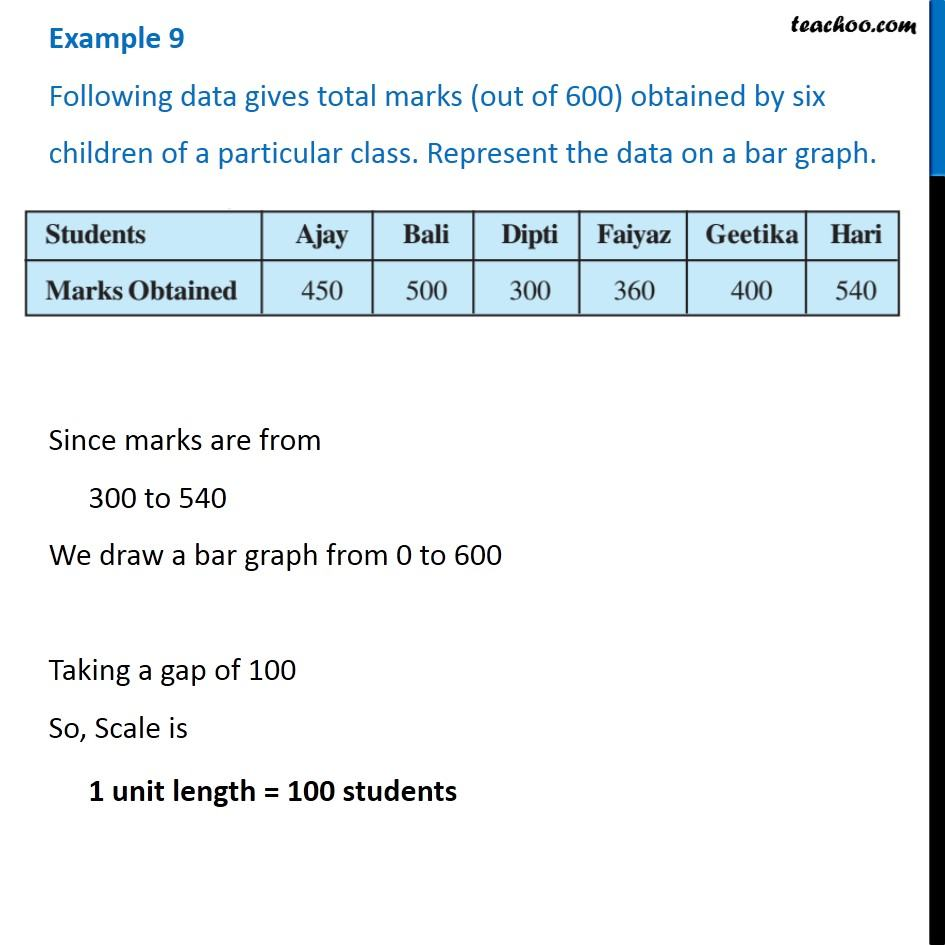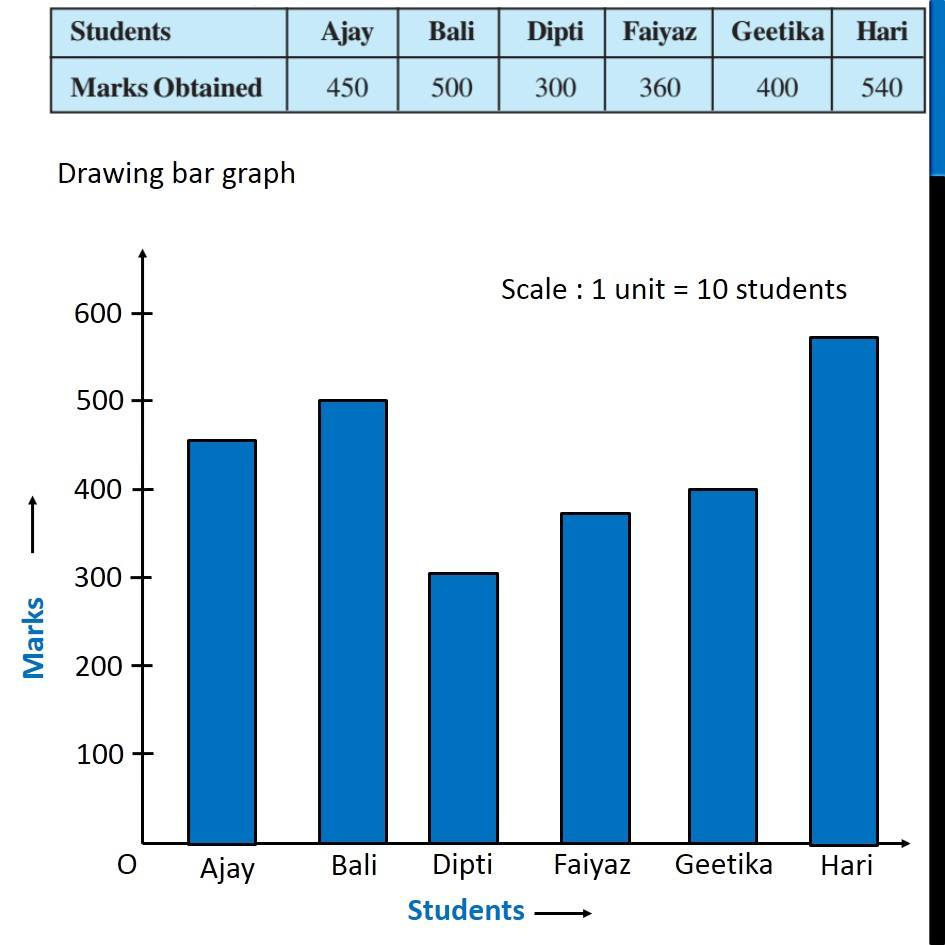Examples

Chapter 3 Class 7 Data Handling
Serial order wise983 students joined Teachoo Black. What are you waiting for?

### Transcript

Example 9 Following data gives total marks (out of 600) obtained by six children of a particular class. Represent the data on a bar graph. Since marks are from 300 to 540 We draw a bar graph from 0 to 600 Taking a gap of 100 So, Scale is 1 unit length = 100 students Drawing bar graph